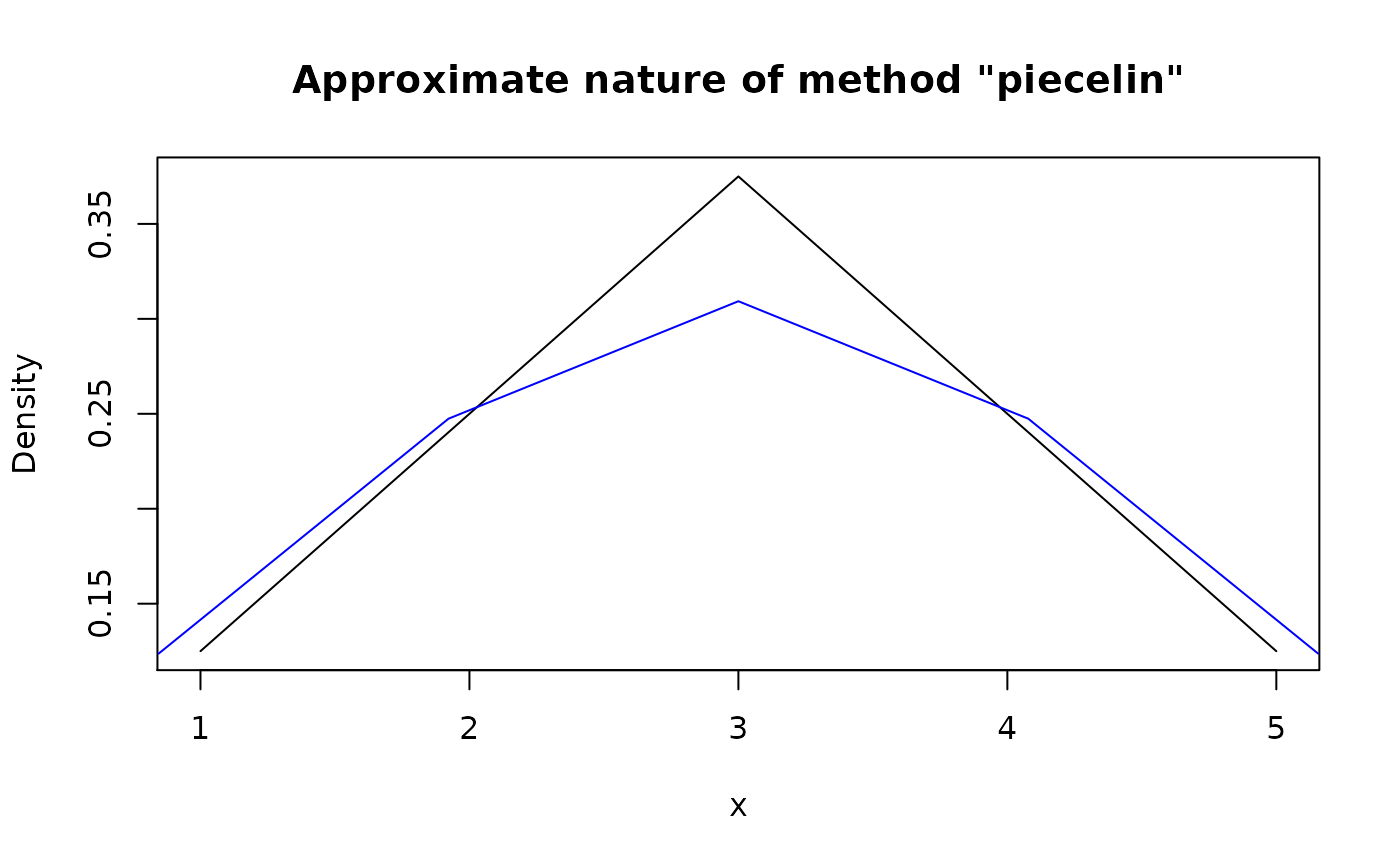Modify type of pdqr-function using method of choice.

form_retype(f, type = NULL, method = "value")

## Arguments

f A pdqr-function. A desired type of output. Should be one of "discrete" or "continuous". If NULL (default), it is chosen as an opposite of f's type. Retyping method. Should be one of "value", "piecelin", "dirac".

## Value

A pdqr-function with type equal to input type.

## Details

If type of f is equal to input type then f is returned.

Method "value" uses renormalized columns of f's "x_tbl" metadata as values for output's "x_tbl" metadata. In other words, it preserves ratios between values of d-function at certain "x" points. Its main advantages are that this method can work well with any pdqr type and that two consecutive conversions return the same function. Conversion algorithm is as follows:

• Retyping from "continuous" to type "discrete" is done by creating pdqr-function of corresponding class with the following "x_tbl" metadata: "x" column is the same as in f; "prob" column is equal to f's "y" column after renormalization (so that their sum is 1).

• Retyping from "discrete" to type "continuous" is done in the same fashion: "x" column is the same; "y" column is equal to f's "prob" column after renormalization (so that total integral of piecewise-linear density is equal to 1).

Method "piecelin" (default) should be used mostly for converting from "continuous" to "discrete" type. It uses the fact that 'pdqr' densities are piecewise-linear (linear in intervals between values of "x" column of "x_tbl" metadata) on their support:

• Retyping from "continuous" to type "discrete" is done by computing "x" values as centers of interval masses with probabilities equal to interval total probabilities.

• Retyping from "discrete" to type "continuous" is made approximately by trying to compute "x" grid, for which "x" values of input distribution are going to be centers of mass. Algorithm is approximate and might result into a big errors in case of small number of "x" values or if they are not "suitable" for this kind of transformation.

Method "dirac" is used mostly for converting from "discrete" to "continuous" type (for example, in form_mix() in case different types of input pdqr-functions). It works in the following way:

• Retyping from "continuous" to type "discrete" works only if "x_tbl" metadata represents a mixture of dirac-like distributions. In that case it is transformed to have "x" values from centers of those dirac-like distributions with corresponding probabilities.

• Retyping from "discrete" to type "continuous" works by transforming each "x" value from "x_tbl" metadata into dirac-like distribution with total probability taken from corresponding value of "prob" column. Output essentially represents a mixture of dirac-like distributions.

form_regrid() for changing grid (rows of "x_tbl" metadata) of pdqr-function.

form_resupport() for changing support of pdqr-function.

Other form functions: form_estimate(), form_mix(), form_regrid(), form_resupport(), form_smooth(), form_tails(), form_trans()

## Examples

my_con <- new_d(data.frame(x = 1:5, y = c(1, 2, 3, 2, 1)/9), "continuous")
meta_x_tbl(my_con)#>   x     y cumprob
#> 1 1 0.125  0.0000
#> 2 2 0.250  0.1875
#> 3 3 0.375  0.5000
#> 4 4 0.250  0.8125
#> 5 5 0.125  1.0000
# By default, conversion is done to the opposite type
my_dis <- form_retype(my_con)
meta_x_tbl(my_dis)#>   x      prob   cumprob
#> 1 1 0.1111111 0.1111111
#> 2 2 0.2222222 0.3333333
#> 3 3 0.3333333 0.6666667
#> 4 4 0.2222222 0.8888889
#> 5 5 0.1111111 1.0000000
# Default retyping (with method "value") is accurate when doing consecutive
# retyping
my_con_2 <- form_retype(my_dis, "continuous")
meta_x_tbl(my_con_2)#>   x     y cumprob
#> 1 1 0.125  0.0000
#> 2 2 0.250  0.1875
#> 3 3 0.375  0.5000
#> 4 4 0.250  0.8125
#> 5 5 0.125  1.0000
# Method "dirac"
my_dirac <- form_retype(my_dis, "continuous", method = "dirac")
meta_x_tbl(my_dirac)#>    x        y    cumprob
#> 1  1        0 0.00000000
#> 2  1 11111111 0.05555556
#> 3  1        0 0.11111111
#> 4  2        0 0.11111111
#> 5  2 22222222 0.22222222
#> 6  2        0 0.33333333
#> 7  3        0 0.33333333
#> 8  3 33333333 0.50000000
#> 9  3        0 0.66666666
#> 10 4        0 0.66666666
#> 11 4 22222222 0.77777777
#> 12 4        0 0.88888888
#> 13 5        0 0.88888888
#> 14 5 11111111 0.94444444
#> 15 5        0 0.99999999
# Method "piecelin"
# From "continuous" to "discrete" (preferred direction)
my_dis_piece <- form_retype(my_con, "discrete", method = "piecelin")
meta_x_tbl(my_dis_piece)#>          x   prob cumprob
#> 1 1.555556 0.1875  0.1875
#> 2 2.533333 0.3125  0.5000
#> 3 3.466667 0.3125  0.8125
#> 4 4.444444 0.1875  1.0000  # Conversion from "discrete" to "continuous" is very approximate
my_con_piece <- form_retype(my_dis_piece, "continuous", method = "piecelin")
meta_x_tbl(my_con_piece)#>           x         y cumprob
#> 1 0.8444444 0.1237113     0.0
#> 2 1.9222222 0.2474227     0.2
#> 3 3.0000000 0.3092784     0.5
#> 4 4.0777778 0.2474227     0.8
#> 5 5.1555556 0.1237113     1.0
plot(my_con, main = 'Approximate nature of method "piecelin"')lines(my_con_piece, col = "blue")